# Regular singular point

(diff) ← Older revision | Latest revision (diff) | Newer revision → (diff)

A notion in the theory of ordinary linear differential equations with an independent complex variable. A pointis called a regular singular point of the equation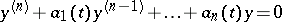(1)

or of the system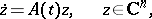(2)

with analytic coefficients, ifis an isolated singularity of the coefficients and if every solution of (1) or (2) increases no faster thanfor some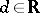, astends towithin an arbitrary acute angle with vertex. This last restriction is necessary in view of the fact that in a neighbourhood of a regular singular point the solutions are non-single-valued analytic functions, and asalong an arbitrary curve, they can increase essentially faster than they do when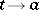over a ray with vertex.

For a singular point of the coefficients of (1) or (2) to be a regular singular point of (1) or (2), it must be a pole (of a function), and not an essential singular point, of the coefficients. For equation (1) there is Fuchs' condition: The singular point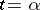of the coefficientsof equation (1) is a regular singular point of (1) if and only if the functions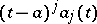,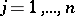, are holomorphic at zero. In the case of the system (2) there is the following sufficient condition: If the entries of the matrixhave a simple pole at a point, then this point is a regular singular point of (2).

How to Cite This Entry:
Regular singular point. Encyclopedia of Mathematics. URL: http://encyclopediaofmath.org/index.php?title=Regular_singular_point&oldid=11770
This article was adapted from an original article by Yu.S. Il'yashenko (originator), which appeared in Encyclopedia of Mathematics - ISBN 1402006098. See original article